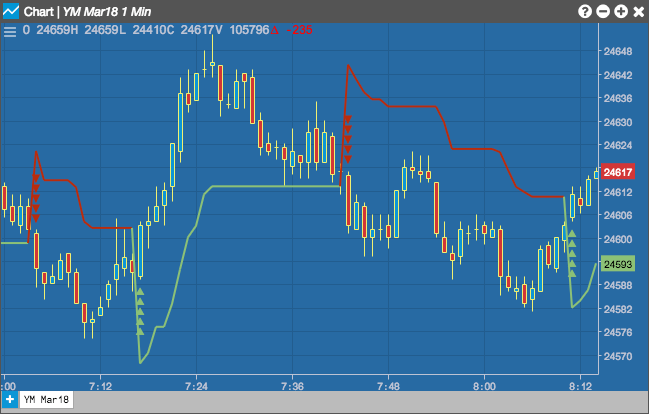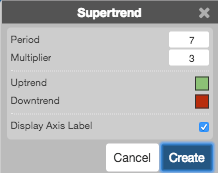Charts

# Supertrend

The Supertrend indicator is used for timing when to buy and sell based on changes in the trend indicator. Typically, a buy signal is when the indicator value closes below the closing price for an instrument, and a sell signal is when the indicator closes above the closing price.## Configuration Options• Period: Number of bars to use in the calculations.
• Mulitplier: Volatility-based mulitplier
• Color Selectors: Colors to use for graph elements.
• Display Axis Label: Whether to display the most recent value on the Y axis.

## Formula

$Upper = \frac{(high + low)}{2} + (Mulitplier * ATR)$

$Lower = \frac{(high + low)}{2} - (Mulitplier * ATR)$